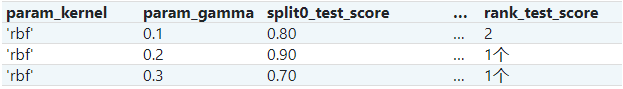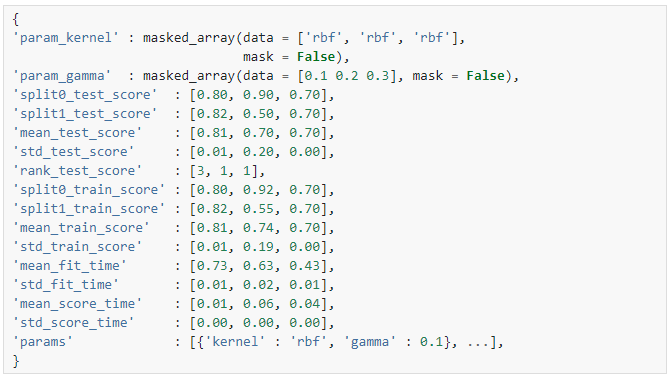# sklearn.model_selection.RandomizedSearchCV¶

class sklearn.model_selection.RandomizedSearchCV（estimator，param_distributions，*，n_iter = 10，评分= None，n_jobs = None，iid ='deprecated'，refit = True，cv = None，verbose = 0，pre_dispatch ='2 * n_jobs'，random_state = None，error_score = nan，return_train_score = False ）

[源码]

RandomizedSearchCV可以实现“fit”和“score”方法。如果在所使用的估计器中实现了“predict”，“predict_proba”，“decision_function”，“transform”和“inverse_transform”，则RandomizedSearchCV也可以实现它们。

estimator estimator object.

param_distributions dict or list of dicts

n_iter int, default=10

scoring str, callable, list/tuple or dict, default=None

n_jobs int, default=None

v0.20版中：n_jobs默认从1更改为None
pre_dispatch int, or str, default=None

-None，在这种情况下，将立即创建并派生所有CPU内核。将此用于轻量级和快速运行的CPU内核，以避免由于按需生成CPU内核而造成的延迟
iid bool, default=False

cv int, cross-validation generator or an iterable, default=None

-None,默认使用5折交叉验证；
-integer,用于指定在(Stratified)KFold中的折叠数；
-CV splitter
- 可迭代输出训练集和测试集的切分作为索引数组

refit bool, str, or callable, default=True

verbose integer

random_state int or RandomState instance, default=None

error_score ‘raise’ or numeric, default=np.nan

return_train_score bool, default=False

cv_results_ dict of numpy (masked) ndarrays用字典cv_results_表示：注意

mean_fit_timestd_fit_timemean_score_timestd_score_time的单位都是秒。

best_estimator_ estimator

best_score_ float
best_estimator的平均交叉验证准确率。

best_params_ dict

best_index_ int

search.cv_results_['params'][search.best_index_]处的字典给出了最优模型的参数设置，该模型给出了最高平均得分（search.best_score_）。

scorer_ function or a dict

n_splits_ int

refit_time_ float

0.20版中的新功能。

GridSearchCV 对参数网格进行详尽搜索。

ParameterSampler 由param_distributions构造的参数设置生成器。

>>> from sklearn.datasets import load_iris>>> from sklearn.linear_model import LogisticRegression>>> from sklearn.model_selection import RandomizedSearchCV>>> from scipy.stats import uniform>>> iris = load_iris()>>> logistic = LogisticRegression(solver='saga', tol=1e-2, max_iter=200,...                               random_state=0)>>> distributions = dict(C=uniform(loc=0, scale=4),...                      penalty=['l2', 'l1'])>>> clf = RandomizedSearchCV(logistic, distributions, random_state=0)>>> search = clf.fit(iris.data, iris.target)>>> search.best_params_{'C': 2..., 'penalty': 'l1'}

decision_function(self, X) 使用找到的最优参数在估计器上调用Decision_function。
fit(self, X[, y, groups]) 将所有参数组合进行拟合。
get_params(self[, deep]) 获取此估计器的参数。
inverse_transform(self, Xt) 用找到的最优参数在估计器上调用inverse_transform。
predict(self, X) 使用找到的最优参数在估计器上调用predict。
predict_log_proba(self, X) 使用找到的最优参数在估计器上调用predict_log_proba。
predict_proba(self, X) 使用找到的最优参数在估计器上调用predict_proba。
score(self, X[, y]) 如果估计器已重新拟合，则返回给定数据的准确率。
set_params(self, **params) 设置此估计器的参数。
transform(self, X) 使用找到的最优参数在估计器上调用transform。
__init__（self，estimator，param_distributions，*，n_iter = 10，得分= None，n_jobs = None，iid =' preprecated '，refit = True，cv = None，verbose = 0，pre_dispatch ='2 * n_jobs'，random_state = None，error_score = nan，return_train_score = False ）

[源码]

decision_function(self, X)

[源码]

X indexable, length n_samples

fit（self，X，y = None，*，groups = None，** fit_params ）

[源码]

X array-like of shape (n_samples, n_features)

y array-like of shape (n_samples, n_output) or (n_samples,), default=None

groups array-like of shape (n_samples,), default=None

**fit_params dict of str -> object

get_params（self，deep = True ）

[源码]

deep bool, default=True

params mapping of string to any

inverse_transform(self, Xt)

[源码]

Xt indexable, length n_samples

predict(self, X)

[源码]

X indexable, length n_samples

predict_log_proba(self, X)

[源码]

X indexable, length n_samples

predict_proba(self, X)

[源码]

X indexable, length n_samples

score(self, X, y=None)

[源码]

X array-like of shape (n_samples, n_features)

y array-like of shape (n_samples, n_output) or (n_samples,), default=None

score float
set_params(self, **params)

[源码]

**params dict

self object

transform(self, X)

[源码]

X indexable, length n_samples

## sklearn.model_selection.RandomizedSearchCV使用示例¶